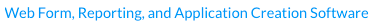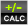RackForms v7.8.8
calculationLatest Release: Build 958 - Arpil 18th, 2023Calculation

In Brief: This module allows us to add all manners of calculation logic to our form fields.

Dependencies: A form page and form fields.

Hints & Tricks:Calculations must always start with a F{form_field_name} = declaration. The code that follows can be pretty much anything we need, just be sure to start a calculation with the F{form_field_name} = bit!

The Calculation module only operates on numeric input and output. That is, the fields we provide to a calculation must be eiether numeric, or a date that's valid when passed though Date.parse(). See more.

Options/Properties

General Usage

Calculation Enabled
Field calculations are enabled by default, though we can disable the calculation action with this control.

### Equation

The heart of the Calculation module is the Equation. It is in this box that we define the fields we wish to perform the logic our final result is based on.

It's important to note that we have shortcuts for adding these fields, which is important as in order for an equation to work the fields we add must be in token format. The good news is the tokens are simple, in that a token is simply the id of the field wrapped in a F{}.

When we define a calculation we MUST specify the result holder first, followed by an equal sign and then the main equation block.

For example, if we have three fields, a, b, and c, with c receiving the result of a + b, we would have the following equation:

F{c} = F{a} + F{b}

Again, the important bit is to make sure the result holder is defined first followed by an equals (=) sign.

### Insert Field List

This field is a convince item for adding equation items. As described above, fields must be wrapped in token format as in F{}. This select item simply lists all fields in a form and allows us to quickly add that item to our Equation field.

Two types of field input are supported: Single Item and Word Count.

Single Item fields are: text-box, radio, select, and checkbox. These items take the raw field value, which must be a number, and use that in our calculation.

Word Count means this field will take the number of individual words in a textarea and use that as our calculation value. This is handy for creating a form that counts words and uses this value to create business logic. For example, a classifieds form may require we count the number of words, and create a total price based on how many words the ad contains.

We identify Word Count fields by the - Word Count text appended to the field name in the item Field List.

Example Word Count Calculation:

F{text3} = F{textarea1} * 10

Here we have a text field, text3, which takes the word count of the textarea1 field, and multiplies the word count by 10. The key here is to note the word count field, textarea1, has no special identifier in the equation, and that we manually entered the multiplication value of * 10.

### Equation Operators:

As with the Insert Field List control, this is a convenience system for adding equation operators.

### Equation Filter

Here we can apply a filter to our equation for things such as currency and decimal places. By default no filter is applied.

Calculation Limitations And General Hints:

• Calculations must start with the receiving field followed by an equal sign.
• For the most complex equations is may be better to write your own custom handler in the Page JavaScript/CSS Code block.
• All fields are processed using the native JavaScript parseFloat() function.
• Only text, select, radio, checkbox, and hidden fields are supported for calculations.
• Only numeric values, or dates that are used in numeric calculations, are allowed as both input and output. Please see the next section for more details.

### Dealing With Non-Numeric Input

The calculations we create can only process numeric values. However, we can process Date values so long as they process down to and are expected to work as output of JavaScript's Date.parse(). A common use of this would be for counting the number of days between two date input values.

For this to work the input must be valid source for the Date.parse() function, which in most browsers means a RFC2822 or ISO 8601 date string. For example:

12/22/1977 or 1977-12-22

Both of these, when fed through Date.parse(), return the proper value of (251622000000), which is the number of seconds since January 1st, 1970.

Note, however, that a slight change to:

12-22-1977 returns NaN, or not a valid number.

This is because that input string is not a valid RFC2822 or ISO 8601 date string.

Please note date calculations are only supported for input field types of: Calendar, Select, Text, and Radio.

### What's Next For Calculations

In future versions of RackForms the Calculation field should be receiving more filters, greater control over updating non-standard fields, and better e-commerce logic support.